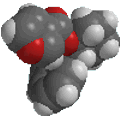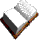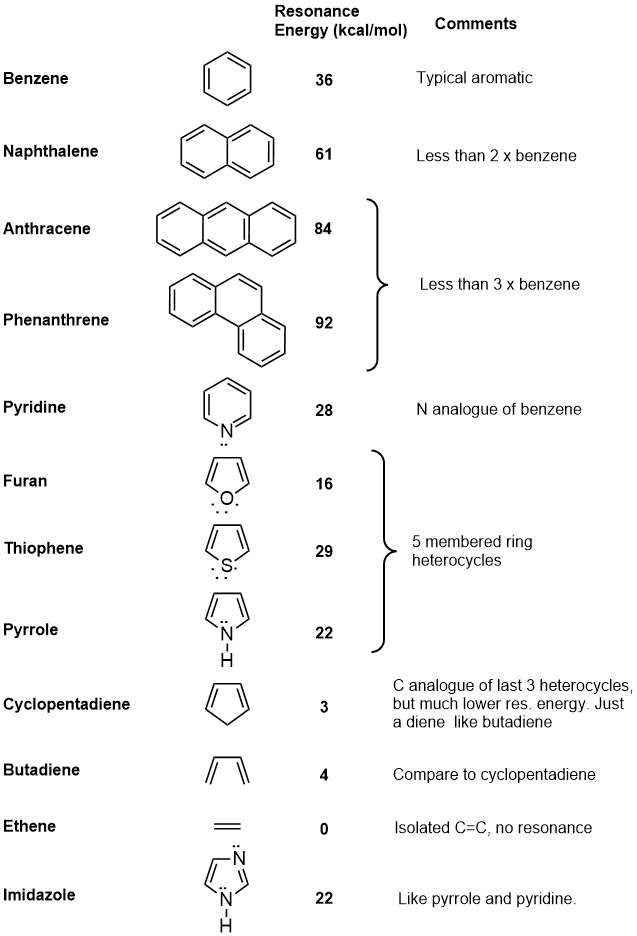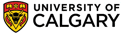Chapter 11 : Arenes and AromaticityResonance Energy

The resonance energy of a compound is a measure of the extra stability of the conjugated system compared to the corresponding number of isolated double bonds.  This can be calculated from experimental measurements.The diagram shows the experimental heats of hydrogenation, DHh, for three molecules, benzene, 1,3-cyclohexadiene and cyclohexene. These are related in that under appropriate conditions that can all be reduced to the same product, cyclohexane.  The DHh for "cyclohexatriene", a hypothetical molecule in which the double bonds are assumed to be isolated from each other, is calculated to be 3 times the value for cyclohexene. This value reflects the energy we could expect to be released from 3 isolated C=C.  By comparing this value with the experimental value for benzene, we can conclude that benzene is 152 kJ or 36 kcal / mol more stable than the hypothetical system. This is the resonance energy for benzene.

QUESTION : Based on the information in the diagram above, what is the resonance energy of 1,3-cyclohexadiene ? ANSWER

 In principle, resonance energies can be calculated for any p systems. The following table contains data on a selection of systems, and some comments about them in relation to benzene or about their aromaticity.© Dr. Ian Hunt, Department of Chemistry